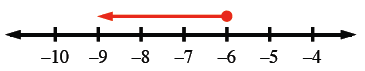### Home > CC2MN > Chapter 9 > Lesson 9.1.3 > Problem9-43

9-43.

Solve and graph the following inequalities:

1. $x-9<17$

• Solve the inequalities like an equation. Substitute the inequality symbol with an equal sign.
$x-9=17$

Isolate the $x$ by adding nine to each side.
$x-9+9=17+9$
$x = 26$

Choose two numbers, one less than $26$ and one greater than $26$. (Example: $3$ and $45$) Substitute these numbers for $x$ to decide which direction the arrow should point.
$x < 26$
$45 < 26$ (False)
$3 < 26$ (True)
Since $3$ is less than $26$, we know that the arrow must point to the left. Every number to the left of $26$ on a number scale is less than $26$.

1. $x + 12 \leq 6$

• Use the same method from part (a).

$x\le-6$1. $10 \geq 4 + x$

• Solve using the same method from part (a).